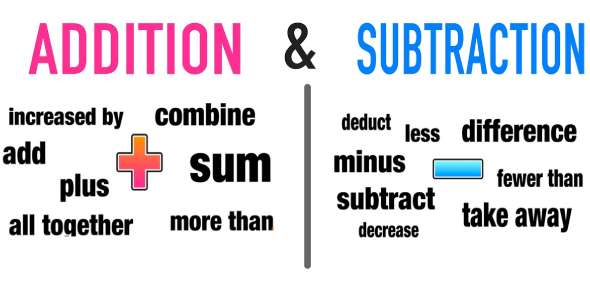# Addition And Subtraction Test: Quiz

10 Questions | Total Attempts: 537SettingsAre you any good at addition and subtraction? Do you think you can pass this quiz? Addition and subtraction are intricately linked. However, addition is the opposite of subtraction. It is also true that every addition and subtraction are connected. Every addition problem can be rewritten as a subtraction problem. Take this quiz and put see if your knowledge about addition and subtraction adds up.

Related Topics
• 1.
3 + 4 =
• A.

7

• B.

8

• C.

1

• D.

9

• 2.
The sum means to what?
• A.

• B.

Subtract

• C.

Multiply

• D.

Fractions

• 3.
6+4 =12
• A.

True

• B.

False

• 4.
7-7 =
• A.

1

• B.

14

• C.

O

• D.

16

• 5.
The difference means to subtract.
• A.

True

• B.

False

• 6.
Find the difference between 6 and 10.
• A.

16

• B.

4

• C.

10

• D.

2

• 7.
Find the sum of 5+5.
• A.

10

• B.

0

• C.

1

• D.

2

• 8.
12-3= 4
• A.

True

• B.

False

• 9.
6+1 =
• A.

7

• B.

5

• C.

8

• D.

10